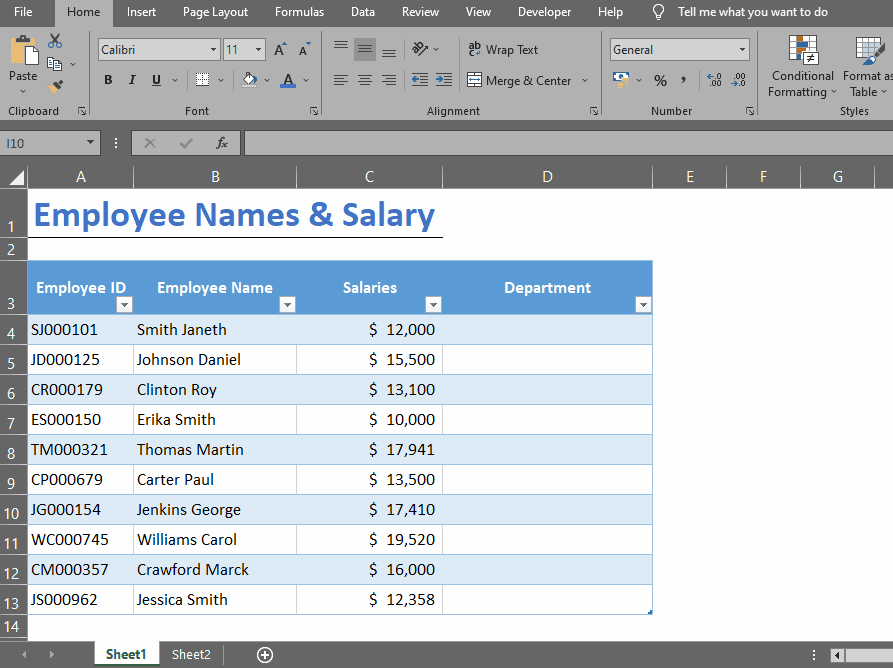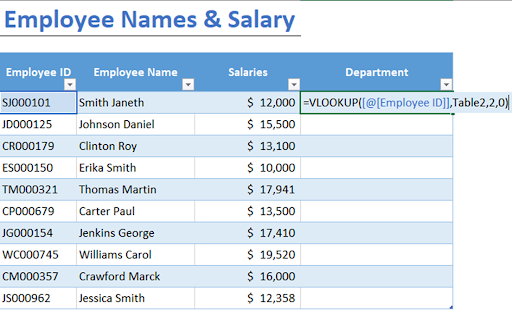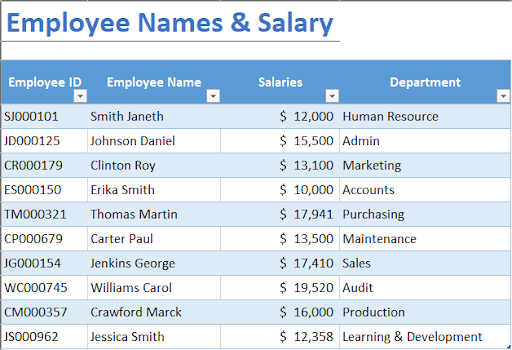# How to join Tables in MS Excel

Table join is a process of combining two or more tables based on a common column. The resulting table contains data from both tables, arranged according to the matching values in the common column. Table join is also known as “data consolidation” or “data merging.” There are several types of table join, each type of join produces a different result, depending on how it matches and combines the data from the two tables. In this article, we will focus on the most common type.

Let’s take a simple example. Suppose we have two tables: Table1 and Table2. Table1 contains a list of employees and their salaries, while Table2 contains a list of employees and their departments. Both tables have a common column, which is “Employee ID.”

To join these tables based on the Employee ID column, we can use the following steps:## Formula BreakdownWhen you have multiple tables of data that you need to combine or analyze, you can join them using Microsoft Excel’s powerful lookup and reference functions. Joining tables can help you create more comprehensive data sets, compare data from different sources, or perform complex calculations.

### Step 1 – Create a New Column In Table 1– Create a new column to hold the Employee Department.

### Step 2 – Type VLOOKUP Formula– Start typing the formula by placing equals to (=) sign.
– Write the following formula in the first cell.
=VLOOKUP([@[Employee ID]], Table2, 2, 0). This will search the matching Employee IDs from table1 with table2 and then fetch the corresponding Department Names.

### Step 3 – Press Enter– Press enter to apply the formula. Now, we have a new column in Table1 that contains the department information for each employee, based on the matching Employee ID in Table2.

### Step 4 – Table Join In MS Excel– Using this new table, we can perform various calculations or create charts and graphs to visualize the data. Table join is a powerful feature of Microsoft Excel that allows you to combine and analyze data from multiple sources.